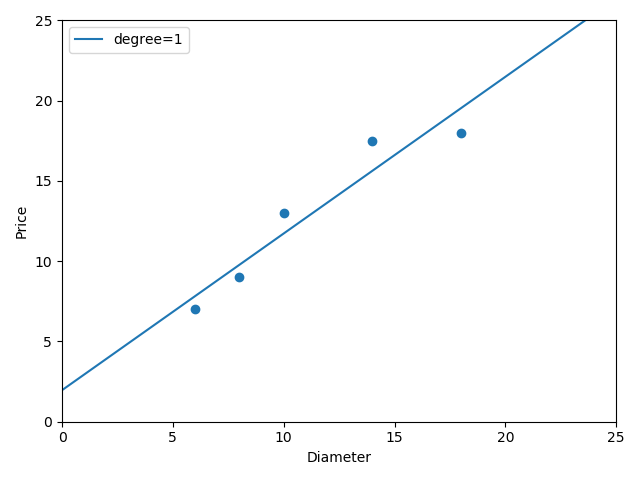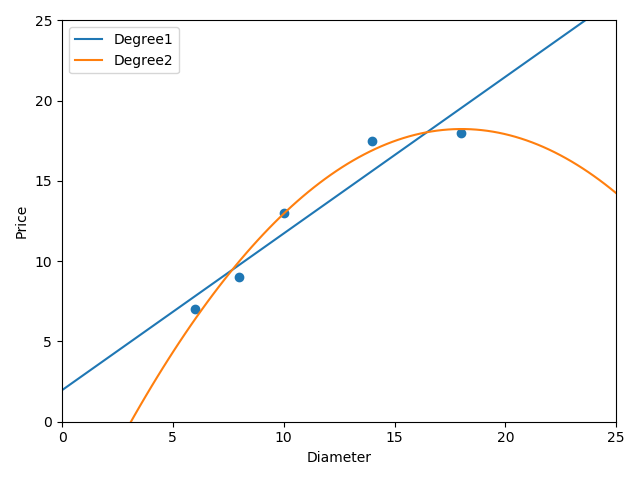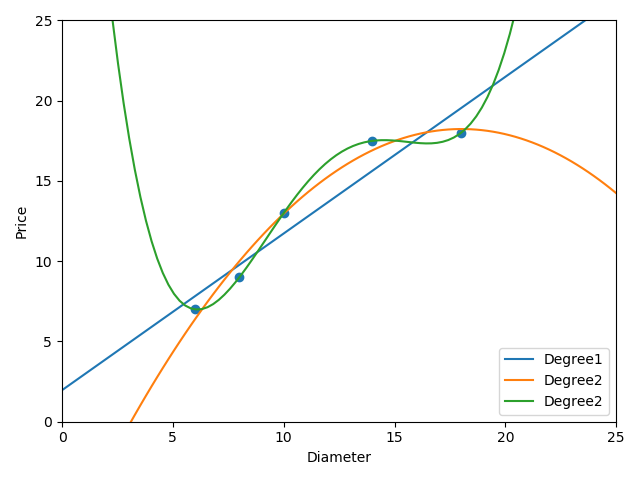# 机器学习之路：python 多项式特征生成PolynomialFeatures 欠拟合与过拟合

分享一下 线性回归中 欠拟合 和 过拟合 是怎么回事~为了解决欠拟合的情 经常要提高线性的次数建立模型拟合曲线， 次数过高会导致过拟合，次数不够会欠拟合。再建立高次函数时候，要利用多项式特征生成器 生成训练数据。下面把整个流程展示一下模拟了一个预测蛋糕价格的从欠拟合到过拟合的过程git: https://github.com/linyi0604/MachineLearning在做线性回归预测时候，为了提高模型的泛化能力，经常采用多次线性函数建立模型f = k*x + b   一次函数f = a*x^2 + b*x + w  二次函数f = a*x^3 + b*x^2 + c*x + w  三次函数。。。泛化：    对未训练过的数据样本进行预测。欠拟合:    由于对训练样本的拟合程度不够，导致模型的泛化能力不足。过拟合：    训练样本拟合非常好，并且学习到了不希望学习到的特征，导致模型的泛化能力不足。在建立超过一次函数的线性回归模型之前，要对默认特征生成多项式特征再输入给模型
　　poly2 = PolynomialFeatures(degree=2)    # 2次多项式特征生成器
x_train_poly2 = poly2.fit_transform(x_train)
下面模拟 根据蛋糕的直径大小 预测蛋糕价格
 1 from sklearn.linear_model import LinearRegression
2 import numpy as np
3 import matplotlib.pyplot as plt
4
5 '''
6 在做线性回归预测时候，
7 为了提高模型的泛化能力，经常采用多次线性函数建立模型
8
9 f = k*x + b   一次函数
10 f = a*x^2 + b*x + w  二次函数
11 f = a*x^3 + b*x^2 + c*x + w  三次函数
12 。。。
13
14 泛化：
15     对未训练过的数据样本进行预测。
16
17 欠拟合:
18     由于对训练样本的拟合程度不够，导致模型的泛化能力不足。
19
20 过拟合：
21     训练样本拟合非常好，并且学习到了不希望学习到的特征，导致模型的泛化能力不足。
22
23
24 在建立超过一次函数的线性回归模型之前，要对默认特征生成多项式特征再输入给模型
25
26 下面模拟 根据蛋糕的直径大小 预测蛋糕价格
27
28 '''
29
30 # 样本的训练数据，特征和目标值
31 x_train = [, , , , ]
32 y_train = [, , , [17.5], ]
33
34 # 一次线性回归的学习与预测
35 # 线性回归模型 学习
36 regressor = LinearRegression()
37 regressor.fit(x_train, y_train)
38 # 画出一次线性回归的拟合曲线
39 xx = np.linspace(0, 25, 100)   # 0到16均匀采集100个点做x轴
40 xx = xx.reshape(xx.shape, 1)
41 yy = regressor.predict(xx)  # 计算每个点对应的y
42 plt.scatter(x_train, y_train)   # 画出训练数据的点
43 plt1, = plt.plot(xx, yy, label="degree=1")
44 plt.axis([0, 25, 0, 25])
45 plt.xlabel("Diameter")
46 plt.ylabel("Price")
47 plt.legend(handles=[plt1])
48 plt.show()1 # 2次线性回归进行预测
2 poly2 = PolynomialFeatures(degree=2)    # 2次多项式特征生成器
3 x_train_poly2 = poly2.fit_transform(x_train)
4 # 建立模型预测
5 regressor_poly2 = LinearRegression()
6 regressor_poly2.fit(x_train_poly2, y_train)
7 # 画出2次线性回归的图
8 xx_poly2 = poly2.transform(xx)
9 yy_poly2 = regressor_poly2.predict(xx_poly2)
10 plt.scatter(x_train, y_train)
11 plt1, = plt.plot(xx, yy, label="Degree1")
12 plt2, = plt.plot(xx, yy_poly2, label="Degree2")
13 plt.axis([0, 25, 0, 25])
14 plt.xlabel("Diameter")
15 plt.ylabel("Price")
16 plt.legend(handles=[plt1, plt2])
17 plt.show()
18 # 输出二次回归模型的预测样本评分
19 print("二次线性模型在训练数据上得分:", regressor_poly2.score(x_train_poly2, y_train))     # 0.98164216395974271 # 进行四次线性回归模型拟合
2 poly4 = PolynomialFeatures(degree=4)    # 4次多项式特征生成器
3 x_train_poly4 = poly4.fit_transform(x_train)
4 # 建立模型预测
5 regressor_poly4 = LinearRegression()
6 regressor_poly4.fit(x_train_poly4, y_train)
7 # 画出2次线性回归的图
8 xx_poly4 = poly4.transform(xx)
9 yy_poly4 = regressor_poly4.predict(xx_poly4)
10 plt.scatter(x_train, y_train)
11 plt1, = plt.plot(xx, yy, label="Degree1")
12 plt2, = plt.plot(xx, yy_poly2, label="Degree2")
13 plt4, = plt.plot(xx, yy_poly4, label="Degree2")
14 plt.axis([0, 25, 0, 25])
15 plt.xlabel("Diameter")
16 plt.ylabel("Price")
17 plt.legend(handles=[plt1, plt2, plt4])
18 plt.show()
19 # 输出二次回归模型的预测样本评分
20 print("四次线性训练数据上得分:", regressor_poly4.score(x_train_poly4, y_train))     # 1.0之前我们一直在展示在训练集合上获得的模型评分，次数越高的模型，训练拟合越好。

1 # 准备测试数据
2 x_test = [, , , ]
3 y_test = [, , , ]
4 print("一次线性模型在测试集合上得分:", regressor.score(x_test, y_test))   # 0.809726797707665
5 x_test_poly2 = poly2.transform(x_test)
6 print("二次线性模型在测试集合上得分:", regressor_poly2.score(x_test_poly2, y_test))   # 0.8675443656345054
7 x_test_poly4 = poly4.transform(x_test)
8 print("四次线性模型在测试集合上得分:", regressor_poly4.score(x_test_poly4, y_test))   # 0.8095880795746723

会发现，二次模型在预测集合上表现最好，四次模型表现反而不好！

01-1410-172939
03-305852
12-144095
12-1044
11-121万+
11-16331
09-03377
11-198万+
01-20515
03-202213
02-14165
08-23436
07-16308
08-24196
10-032906
05-021688点击重新获取扫码支付余额充值